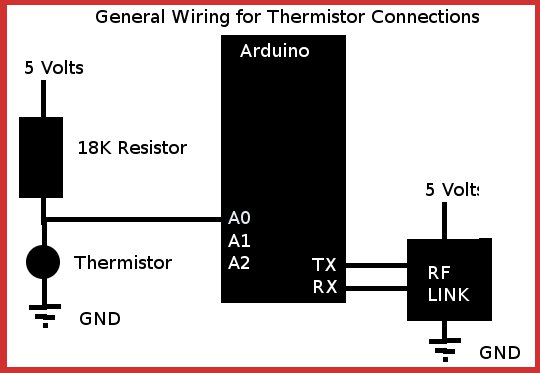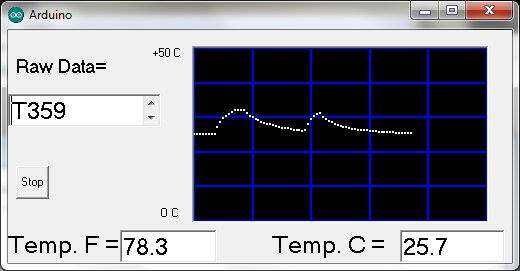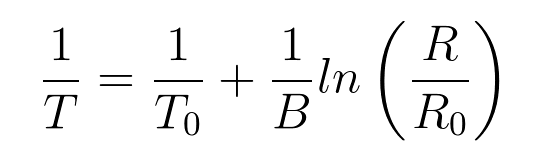# Using a Thermistor, RF Link, and Arduino to Report Temperature

The initial program simply reported back to the serial monitor about 300 feet away in our house at 1 second intervals. A serial RF link was attached to the arduino via the TX and RX connections. (The program has to be loaded before the RF link is connected, otherwise you can't download the program to the Arduino.) The APC220 RF link was used. In it's simplest form this is a 440 MHZ link (documented on net). No attempt was made to change the out of the box defaults.Similar to this version at DX.COM. The Beta parameter equation is used here to calculate temperature in Fahrenheit and Centigrade. General reference To do this, the series resistance equation was used to calculate the resistance of the thermistor. R1/(R1+R2)*Vin=Vout
This can be rewritten as R1/(R1+18000)*1023=count, where count is the output from the A/D converter, Divide both sides by 1023 where k=count/1023 then with R2=18000, This means R1=k*18000/(1- k). And yes dear Horatio, you can argue that there for that 0-5V 10 bit A to D converter that there are 1024 levels but the span is only 1023 not 1024, i.e. 5V is 1023 not 1024 as most authors assume.
 ``` //July 8, 2015 //Redo of an earlier TI MSP430 Launchpad program, this VB program uses A0 input on an Arduino to monitor a thermistor. //in simple series with an 18k resistor. Raw data represents output from 10 bit A/D (0 to 1023). //Thermistor on Ebay: 100pcs 10k OHM Thermistor Resistor NTC-MF52-103J/3435 10K ±5% 3435±1% , \$5.00 //Thermistor cost \$0.05... //========First Arduino program =========== int a=0; void setup() {Serial.begin(9600); } void loop(){ a=analogRead(0); Serial.print("T"); Serial.println(a); delay(1000); }`````` 'MSP430 and Arduino VB-6 serial communications program '--------------------------------------------- Dim w, temp Private Sub Command1_Click() 'Turn on or off using push button If MSComm1.PortOpen = False Then Command1.Caption = "Stop" MSComm1.PortOpen = True 'launch receive subroutine Call probie Else Command1.Caption = "Go" MSComm1.PortOpen = False Text1.Text = "---OFF---" End If End Sub '--------------------------------------------- Private Sub Form_Activate() 'Initialize the serial port.... t = 0 Picture1.Scale (0, 50)-(100, 0): Picture1.ForeColor = vbBlue For s = 0 To 100 Step 20: Picture1.Line (s, 0)-(s, 50): Next For s = 0 To 50 Step 10: Picture1.Line (0, s)-(100, s): Next : Picture1.ForeColor = vbWhite While Val(cp) = 0 cp = InputBox("Comm Port#", "Com#", "5") Wend MSComm1.RThreshold = 1 ' When Inputting Data, Input 1 Byte at a time MSComm1.InputLen = 1 ' 2400 Baud, No Parity, 8 Data Bits, 1 Stop Bit (set to same baud rate as Java Gui) MSComm1.Settings = "9600,N,8,1" ' Disable DTR MSComm1.DTREnable = False ' Open COM3 ===== Check Com port by opening devices in control panel/Yours may differ MSComm1.CommPort = Val(cp) MSComm1.PortOpen = True ' ================================= 'launch receive subroutine Call probie End Sub '--------------------------------------------- Sub probie() 'Recieve subroutine - Wait for transmitted packets from shop - (no error check for packet drop) While MSComm1.PortOpen = True ' If comEvReceive Event then get data and display If MSComm1.CommEvent = comEvReceive Then Data = MSComm1.Input 'grab byte if port active If Data = "T" Then Text1.Text = "": woof = 1 'Display Temperature If Data <> "" Then Text1.Text = Text1 + Data 'Display Temperature ' Find w, which is the thermistor resistance, by using the series resistance formula If Len(Text1.Text) = 4 And woof = 1 Then r = Val(Right\$(Text1.Text, 3)): k = r / 1023: w = k * 18000 / (1 - k): burko Picture1.PSet (t, temp - 273): t = t + 1: If t > 99 Then t = 0 woof = 0 End If End If DoEvents 'Don't be too busy to check push button :-) Wend End Sub '--------------------------------------------- 'Private Sub Form_Unload(Cancel As Integer) 'close the form End End Sub '--------------------------------------------- Sub burko() 'This subroutine scales the thermistor curve to give F and C data. Beta=3435, For a different thermistor, ' use a different Beta value and center resistance value, 10k ohms at 298 Kelvin 'Uses the Beta parameter equation (see Wikipedia) to linearize the thermistor output and calculate the temperature ntemp = 1 / 298 + (1 / 3435) * Log(w / 10000) temp = 1 / ntemp: C = temp - 273 :'find and convert Kelvin to centigrade Text2.Text = Format(C, "###.#"): F = (9 / 5) * C + 32 Text3.Text = Format(F, "###.#") :'find and convert Centigrade to Fahrenheit End Sub```But what if I want the house to poll the greenhouse, outdoors, and shop for three different values? Then do this:``` //Arduino program for remote polling of 3 thermistors const int switchPin=3; const int ledPin=13; int a=0; int switchState=0; int sv=0; //=====setup========== void setup(){ pinMode(ledPin,OUTPUT); pinMode(switchPin,INPUT); Serial.begin(9600); switchState=digitalRead(switchPin);} //=====Program Loop============= void loop(){ sv=Serial.read(); //Tell me which thermistor data to send // digitalWrite(ledPin,HIGH);delay(100); // digitalWrite(ledPin,LOW);delay(100); switch(sv){ case'0': Serial.print("T"); a=analogRead(0); Serial.println(a);//put 10 ms delay here digitalWrite(ledPin,HIGH);delay(300); digitalWrite(ledPin,LOW);delay(300); break; case '1': Serial.print("U"); a=analogRead(1); Serial.println(a);//put 10 ms delay here digitalWrite(ledPin,HIGH);delay(300); digitalWrite(ledPin,LOW);delay(300); digitalWrite(ledPin,HIGH);delay(300); digitalWrite(ledPin,LOW);delay(300); break; case '2': Serial.print("V"); a=analogRead(2); Serial.println(a);//put 10 ms delay here digitalWrite(ledPin,HIGH);delay(300); digitalWrite(ledPin,LOW);delay(300); digitalWrite(ledPin,HIGH);delay(300); digitalWrite(ledPin,LOW);delay(300); digitalWrite(ledPin,HIGH);delay(300); digitalWrite(ledPin,LOW);delay(300); break; } delay(100);} ```

The VB-6 program is shown below... VB6 beats VB.net for the same reason that Arduino beats TI Launchpad... simplicity!!
 ``` 'VB-6 Program for three thermistors as polled from home base with some error processing Dim w, temp, q, t, da(1440), foo, dpks, data As String, dsource, ghu, ghl, shu, shl '---------------------------------------------------------- Private Sub Command1_Click() 'Turn on or off using push button If MSComm1.PortOpen = False Then Timer1.Enabled = True Command1.Caption = "Stop" MSComm1.PortOpen = True 'launch receive subroutine Call probie Else Timer1.Enabled = False Command1.Caption = "Go" MSComm1.PortOpen = False Text1.Text = "---OFF---" End If End Sub '---------------------------------------------------------- Private Sub Command2_Click() If Timer1.Enabled = True Then Timer1.Enabled = False Beep Picture1.Cls DoEvents Call pokadot For num = 0 To 1440 Picture1.PSet (num, da(num)) Next num End Sub '---------------------------------------------------------- Private Sub Command3_Click() If MSComm1.PortOpen = False Then MSComm1.PortOpen = True Call probie End Sub '---------------------------------------------------------- Private Sub Form_Activate() 'Initialize the serial port.... t = 0 ghu = 80 ghl = 60 shu = 80 shl = 60 Image1(0).Visible = False Image1(1).Visible = False Image1(2).Visible = False Image1(3).Visible = False Call pokadot DoEvents cp = 0 While Val(cp) = 0 cp = InputBox("Comm Port#", "Com#", "10") Wend MSComm1.RThreshold = 1 ' When Inputting Data, Input 1 Byte at a time MSComm1.InputLen = 1 ' 9600 Baud, No Parity, 8 Data Bits, 1 Stop Bit MSComm1.Settings = "9600,N,8,1" ' Disable DTR & RTS MSComm1.DTREnable = False ' MSComm1.RTSEnable = False ' Open COM3 ===== Check Com port by opening devices in control panel/Yours may differ MSComm1.CommPort = Val(cp) If MSComm1.PortOpen = False Then MSComm1.PortOpen = True ' ================================= t = 60 * Hour(Time) + Minute(Time) x = 6 Label9.Caption = "Started: " & Time & " " & Date & " " & InputBox("Location:", "Where") 'launch receive subroutine Timer1.Enabled = True End Sub '---------------------------------------------------------- Private Sub Form_Unload(Cancel As Integer) 'close the form End End Sub '---------------------------------------------------------- Sub burko() ntemp = 1 / 298 + (1 / 3435) * Log(w / 10000) temp = 1 / ntemp: C = temp - 273 If dsource = 0 Then Text3.Text = Format(C, "###.#"): f = (9 / 5) * C + 32 Text2.Text = Format(f, "###.#") If f < Val(ghl) Then Image1(0).Visible = True Else Image1(0).Visible = False If f > Val(ghu) Then Image1(1).Visible = True Else Image1(1).Visible = False ElseIf dsource = 1 Then Text7.Text = Format(C, "###.#"): f = (9 / 5) * C + 32 Text6.Text = Format(f, "###.#") If f < Val(shl) Then Image1(2).Visible = True Else Image1(2).Visible = False If f > Val(shu) Then Image1(3).Visible = True Else Image1(3).Visible = False ElseIf dsource = 2 Then Text10.Text = Format(C, "###.#"): f = (9 / 5) * C + 32 Text9.Text = Format(f, "###.#") End If End Sub '---------------------------------------------------------- Private Sub Text11_Click() ghu = InputBox("Enter Greenhouse Cooling Set Point", , ghu): Text11.Text = ghu End Sub '---------------------------------------------------------- Private Sub Text12_Click() shl = InputBox("Enter Shop Heating Set Point", , shl): Text12.Text = shl End Sub '---------------------------------------------------------- Private Sub Text13_click() shu = InputBox("Enter Shop Cooling Set Point", , shu): Text13.Text = shu End Sub '---------------------------------------------------------- Private Sub Text8_Click() ghl = InputBox("Enter Heating Set Point", , ghl): Text8.Text = ghl End Sub '---------------------------------------------------------- Private Sub Timer1_Timer() DoEvents Label6 = "Min.:" & t & ", Time:" & Hour(Time) & ":" & Minute(Time) q = q + 1: Text1.Text = q If q = 60 Then q = 0 End If If q = 0 Then Call probie If q = 20 Then Call gobi If q = 40 Then Call mobie t = 60 * Hour(Time) + Minute(Time) End Sub '---------------------------------------------------------- Sub pokadot() Picture1.Scale (0, 50)-(1440, -40): Picture1.BackColor = RGB(80, 0, 0): Picture1.Cls Picture1.Line (0, 20)-(1440, 30), RGB(0, 80, 0), BF Picture1.Line (0, -40)-(1440, 20), RGB(0, 0, 80), BF Picture1.ForeColor = vbGreen For s = 120 To 1440 Step 120: Picture1.Line (s, -40)-(s, 50): Next For s = -40 To 50 Step 10: Picture1.Line (0, s)-(1440, s): Next : Picture1.ForeColor = vbWhite End Sub '---------------------------------------------------------- Sub probie() 'Recieve subroutine dsource = 0 foo = 2000: data = "": Text1.Text = "": r = 0: MSComm1.InBufferCount = 0: MSComm1.Output = "0" & vbCrLf While MSComm1.PortOpen = True foo = foo - 1 ' If comEvReceive Event then get data and display If MSComm1.CommEvent = comEvReceive Then data = MSComm1.Input 'grab byte if port active If data = "T" Then Text1.Text = "": woof = 1 If data = vbCr Then Beep 'Display Temperature If data <> "" Then Text1.Text = Text1 + data 'Display Temperature If Len(Text1.Text) >= 4 And woof = 1 Then r = Val(Mid\$(Text1.Text, 2, 3)): k = r / 1023: w = k * 18000 / (1 - k): burko Picture1.PSet (t, temp - 273), vbWhite: da(t) = temp - 273 woof = 0: data = "": Exit Sub End If End If If foo = 0 Then dpks = dpks + 1: Text4.Text = dpks: Exit Sub DoEvents 'Don't be too busy to check push button :-) ss = ss + 1: Text5.Text = ss Wend End Sub '---------------------------------------------------------- Sub mobie() dsource = 1 foo = 2000: data = "": Text1.Text = "": r = 0: MSComm1.InBufferCount = 0: MSComm1.Output = "1" & vbCrLf While MSComm1.PortOpen = True foo = foo - 1 ' If comEvReceive Event then get data and display If MSComm1.CommEvent = comEvReceive Then data = MSComm1.Input 'grab byte if port active If data = "U" Then Text1.Text = "": woof = 1 If data = vbCr Then Beep 'Display Temperature If data <> "" Then Text1.Text = Text1 + data 'Display Temperature If Len(Text1.Text) >= 4 And woof = 1 Then r = Val(Mid\$(Text1.Text, 2, 3)): k = r / 1023: w = k * 18000 / (1 - k): burko Picture1.PSet (t, temp - 273), vbYellow: da(t) = temp - 273 woof = 0: data = "": Exit Sub End If End If If foo = 0 Then dpks = dpks + 1: Text4.Text = dpks: Exit Sub DoEvents 'Don't be too busy to check push button :-) ss = ss + 1: Text5.Text = ss Wend End Sub ---------------------------------------------- Sub gobi() dsource = 2 foo = 2000: data = "": Text1.Text = "": r = 0: MSComm1.InBufferCount = 0: MSComm1.Output = "2" & vbCrLf While MSComm1.PortOpen = True foo = foo - 1 ' If comEvReceive Event then get data and display If MSComm1.CommEvent = comEvReceive Then data = MSComm1.Input 'grab byte if port active If data = "V" Then Text1.Text = "": woof = 1 If data = vbCr Then Beep 'Display Temperature If data <> "" Then Text1.Text = Text1 + data 'Display Temperature If Len(Text1.Text) >= 4 And woof = 1 Then r = Val(Mid\$(Text1.Text, 2, 3)): k = r / 1023: w = k * 18000 / (1 - k): burko Picture1.PSet (t, temp - 273), RGB(255, 130, 130): da(t) = temp - 273 woof = 0: data = "": Exit Sub End If End If If foo = 0 Then dpks = dpks + 1: Text4.Text = dpks: Exit Sub DoEvents 'Don't be too busy to check push button :-) ss = ss + 1: Text5.Text = ss Wend End Sub ```

Data sheets and arduino connections to the APC220 V3 RF module can be found online. There are two subroutines that compute the temperature and this could be done at the arduino by using the math.h include file. Instead, the analog to digital converter supplies and transmits a number between 0 and 1023. This represents in a linear way, the resistor ratio: R1/(R1+R2) which varies between 0 and 1, where R1 is the thermistor resistance, and R2 is selected arbitrarily to give maximum change at the temperature of interest (slightly below room temperature). R2 was selected as 18,000 ohms partly because this was close to the desired thermistor optimum somewhat below room temp. and partly because I had some high precision resistors in my toolbox with this value. So, if I divided the incoming data by 1023, I got the ratio R1/(R!+R2). You'll find this equation in the subroutine "probie." k=r/1023. Step one was to scale the analog reading to a value between zero and one.
Next set:

k=R1/(R1+18,000) and solve for R1.

k*R1+k*18,000=R1

k*18000=R1-k*R1

k*18,000=R1(1-k) ....or

R1=18000* k/(1-k) .... this is the thermistor resistance read by the arduino.
An arduino with higher resolution is available (12 bit), Arduino Due?? The Beta parameter equation then lets you calculate temperature. The beta value is usually listed when the thermistor is sold, very convenient. This is shown here as a photo from Wikipedia and appears in the subroutine "burko." It lets almost any thermistor to be read simply and without any sweat whatsoever, just a matter of typing carefully :) To get the resolution at a particular temperature, arbitrarily change the analog value by one unit, and then recalculate the temperature, the difference between that temperature and the read value is the temperature resolution. Pretty good, to be honest, tenths or hundredths of a degree usually. Some self heating occurs due to current flow. This can throw off the readings but my experience is that this is usually small and can be compensated for. .Here it is about 1.8 mW/degree Celsius ( https://www.mouser.com/datasheet/2/427/ntcle213e3-1762456.pdf ). Consult thermistor data sheets for this value under different insulation and/or air flow conditions... At 18k the power dissipated would be about P=V^2/R=6.25/18000 . And power would be I^2*R or an error of about 0.2 degrees C in still air.
Thanks for your attention, sincerely, Me....

That's all folks, but if you can put stuff down here that you don't want anyone to read.